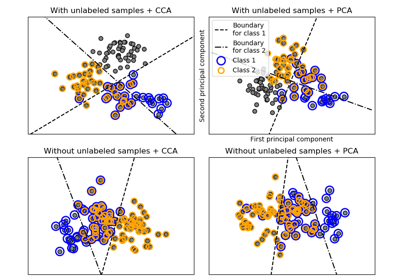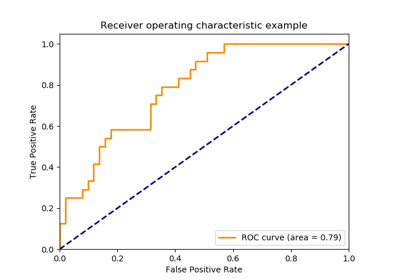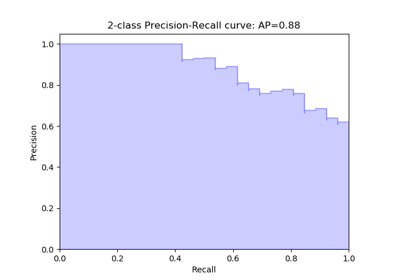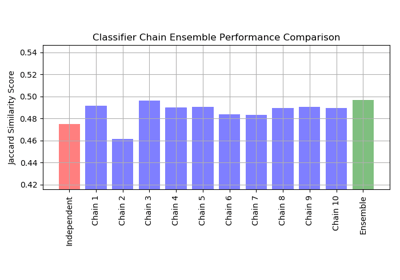/scikit-learn

# sklearn.multiclass.OneVsRestClassifier

`class sklearn.multiclass.OneVsRestClassifier(estimator, n_jobs=None)` [source]

One-vs-the-rest (OvR) multiclass/multilabel strategy

Also known as one-vs-all, this strategy consists in fitting one classifier per class. For each classifier, the class is fitted against all the other classes. In addition to its computational efficiency (only `n_classes` classifiers are needed), one advantage of this approach is its interpretability. Since each class is represented by one and one classifier only, it is possible to gain knowledge about the class by inspecting its corresponding classifier. This is the most commonly used strategy for multiclass classification and is a fair default choice.

This strategy can also be used for multilabel learning, where a classifier is used to predict multiple labels for instance, by fitting on a 2-d matrix in which cell [i, j] is 1 if sample i has label j and 0 otherwise.

In the multilabel learning literature, OvR is also known as the binary relevance method.

Read more in the User Guide.

Parameters: `estimator : estimator object` An estimator object implementing `fit` and one of `decision_function` or `predict_proba`. `n_jobs : int or None, optional (default=None)` The number of jobs to use for the computation. `None` means 1 unless in a `joblib.parallel_backend` context. `-1` means using all processors. See Glossary for more details. `estimators_ : list of n_classes estimators` Estimators used for predictions. `classes_ : array, shape = [n_classes]` Class labels. `label_binarizer_ : LabelBinarizer object` Object used to transform multiclass labels to binary labels and vice-versa. `multilabel_ : boolean` Whether this is a multilabel classifier

#### Methods

 `decision_function`(X) Returns the distance of each sample from the decision boundary for each class. `fit`(X, y) Fit underlying estimators. `get_params`([deep]) Get parameters for this estimator. `partial_fit`(X, y[, classes]) Partially fit underlying estimators `predict`(X) Predict multi-class targets using underlying estimators. `predict_proba`(X) Probability estimates. `score`(X, y[, sample_weight]) Returns the mean accuracy on the given test data and labels. `set_params`(**params) Set the parameters of this estimator.
`__init__(estimator, n_jobs=None)` [source]
`decision_function(X)` [source]

Returns the distance of each sample from the decision boundary for each class. This can only be used with estimators which implement the decision_function method.

Parameters: `X : array-like, shape = [n_samples, n_features]` `T : array-like, shape = [n_samples, n_classes]`
`fit(X, y)` [source]

Fit underlying estimators.

Parameters: `X : (sparse) array-like, shape = [n_samples, n_features]` Data. `y : (sparse) array-like, shape = [n_samples, ], [n_samples, n_classes]` Multi-class targets. An indicator matrix turns on multilabel classification. self
`get_params(deep=True)` [source]

Get parameters for this estimator.

Parameters: `deep : boolean, optional` If True, will return the parameters for this estimator and contained subobjects that are estimators. `params : mapping of string to any` Parameter names mapped to their values.
`multilabel_`

Whether this is a multilabel classifier

`partial_fit(X, y, classes=None)` [source]

Partially fit underlying estimators

Should be used when memory is inefficient to train all data. Chunks of data can be passed in several iteration.

Parameters: `X : (sparse) array-like, shape = [n_samples, n_features]` Data. `y : (sparse) array-like, shape = [n_samples, ], [n_samples, n_classes]` Multi-class targets. An indicator matrix turns on multilabel classification. `classes : array, shape (n_classes, )` Classes across all calls to partial_fit. Can be obtained via `np.unique(y_all)`, where y_all is the target vector of the entire dataset. This argument is only required in the first call of partial_fit and can be omitted in the subsequent calls. self
`predict(X)` [source]

Predict multi-class targets using underlying estimators.

Parameters: `X : (sparse) array-like, shape = [n_samples, n_features]` Data. `y : (sparse) array-like, shape = [n_samples, ], [n_samples, n_classes].` Predicted multi-class targets.
`predict_proba(X)` [source]

Probability estimates.

The returned estimates for all classes are ordered by label of classes.

Note that in the multilabel case, each sample can have any number of labels. This returns the marginal probability that the given sample has the label in question. For example, it is entirely consistent that two labels both have a 90% probability of applying to a given sample.

In the single label multiclass case, the rows of the returned matrix sum to 1.

Parameters: `X : array-like, shape = [n_samples, n_features]` `T : (sparse) array-like, shape = [n_samples, n_classes]` Returns the probability of the sample for each class in the model, where classes are ordered as they are in `self.classes_`.
`score(X, y, sample_weight=None)` [source]

Returns the mean accuracy on the given test data and labels.

In multi-label classification, this is the subset accuracy which is a harsh metric since you require for each sample that each label set be correctly predicted.

Parameters: `X : array-like, shape = (n_samples, n_features)` Test samples. `y : array-like, shape = (n_samples) or (n_samples, n_outputs)` True labels for X. `sample_weight : array-like, shape = [n_samples], optional` Sample weights. `score : float` Mean accuracy of self.predict(X) wrt. y.
`set_params(**params)` [source]

Set the parameters of this estimator.

The method works on simple estimators as well as on nested objects (such as pipelines). The latter have parameters of the form `<component>__<parameter>` so that it’s possible to update each component of a nested object.

Returns: self

## Examples using `sklearn.multiclass.OneVsRestClassifier`Multilabel classificationReceiver Operating Characteristic (ROC)Precision-RecallClassifier Chain

© 2007–2018 The scikit-learn developers